Fourier Cosine Series

## Linear Methods of Applied Mathematics Evans M. Harrell II and James V. Herod*

version of 26 December 1995

There are three distinct versions of the Fourier series in common use, the "full" Fourier series, the Fourier sine series, and the Fourier cosine series. They are based on slightly different choices of an orthogonal basis set, whether (2.6) for the Fourier sine series, (2.7) for the Fourier cosine series, or (2.8) for the full Fourier series. Notice that while the full set (2.8) contains both sines and cosines, it does not contain as many frequencies as either of the other sets, since the trig functions depend on 2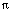n x/L rather than all integer multiples ofx/L. We shall also discuss an "exponential" Fourier series, which uses complex functions of the form

exp( 2i x/L),

but this is actually the same series as the full Fourier serie: It has simply been written in a convenient way using exponential functions.

The three different sets do an equally good job of approximating functions in the r.m.s. sense, but their relationship to symmetries is different. For this reason, different sets of Fourier functions will arise in our study of partial differential equations, depending on which kind of "boundary conditions" which are physically appropriate for a given problem.

The full Fourier series consists of functions which are periodic , and are particularly good for representing or approximating periodic functions, that is, functions for which f(x + L) = f(x). Examples include sounds built on a basic frequency with overtones or a physical function depending on an angular variable such as longitude. The Fourier cosine series, in contrast, is well adapted to functions which have zero derivatives at x=0 and x=L, since all the functions cos(nx/L) have this property. A related but less obvious property that begs for the use of cosine functions is an even symmetry: f(-x) = f(x). If the function you wish to approximate is an even function, then the cosines are a good candidate for a basis set. Notice that the cosines are not only even under reflection about 0, but also about the other end of the interval, x=L. Fourier sine series are better suited for odd functions. A Fourier cosine series represents a function as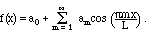x/L)">     (3.b1)
for 0 < x < L. In most applications a = 0 < x < L, but this is not strictly necessary. If the lower limit for x is a > 0, then the Fourier cosine series can still be used if we change variables from x to x-a, so the basis functions are cos (m pi (x-a)/L).

The formulae for the coefficients a_m are the same as in (2.12) and (2.13), except that the Fourier cosine series uses all integer multiples ofx/L, where L=b-a, not just the even multiples: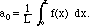while for m>0,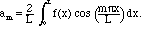x/L)">     (3.b2)
Just as for the full Fourier series, the Fourier sine functions are a complete set, and they satisfy some useful identities, which I have collected in a theorem (which is almost identical to the one for the full series). Historically, parts of this theorem were contributed by Fourier, Parseval, Plancherel, Riesz, and Fischer, and in most sources it is considered several theorems. For proofs and further details, refer to Rudin's Real Analysis  or Rogosinski's Fourier Series .

The powerful theorem behind the Fourier cosine series III.3b.

If f(x) is square-integrable on an interval [a,b], then

a) All of the coefficients am of (3.b2) are well defined.

b) All of the coefficients am are uniquely determined by f(x) and depend linearly on f(x).

b) The series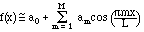converges to f(x) in the mean-square sense. In other words,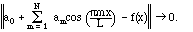We shall express this by writing(Remember, however, that this series converges in a mean sense, and not necessarily at any given point.)

d)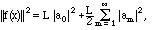and the right side is guaranteed to converge.

e) If g is a second square-integrable function, with Fourier cosine coefficients a-tilde, then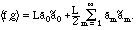This is known as the Parseval formula  for the Fourier cosine series.

Conversely, given a square-summable sequence a_m, i.e., real or complex numbers such that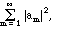is finite, they determine a square integrable function f(x) uniquely a.e. such that all the statements of this theorem hold.

In addition, if f(x) is piecewise continuous, then Theorem III.6 likewise holds, except at the end points of the interval. If 0 < x < L, the Fourier cosine series will converge to the average of the right and left limiting values of f at x. When x=0 or L, however, the Fourier cosine series will always converge to the one-sided limit of f at that point.

When we study partial differential equations beginning in chapter VI, Fourier sine or cosine functions will arise depending on the boundary conditions. If we know that the functions we seek are equal to 0 at the end points 0 and L, then we can ensure this fact by writing them as sums of sines of the form sin(nx/L). Similarly, if we know that the derivatives are equal to 0 at the end points, we can accomplish this with a sum of cosines of the form cos(mx/L).

Compare with the Fourier sine series

Compare with the full Fourier series

Compare with the exponential Fourier series